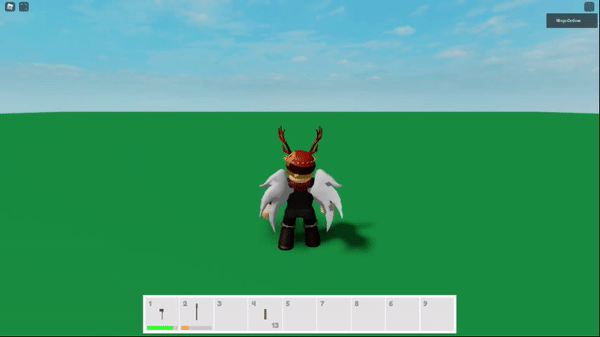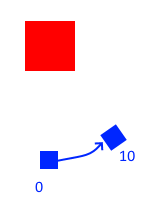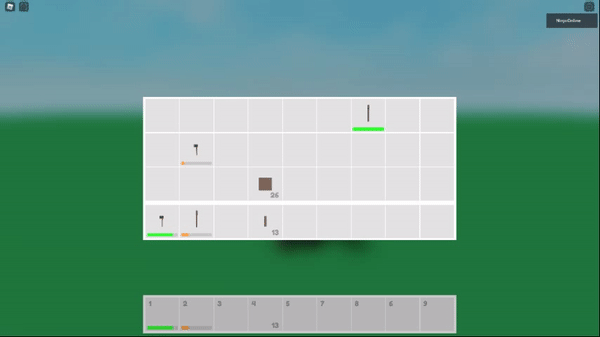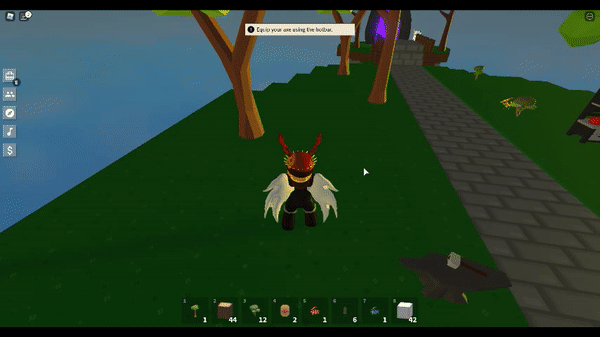# Camera not rotating around a model pivet correctly?

I want to rotate my camera slightly when you hover over an item, but atm it does this like full circle motion.``````local Offset = CFrame.new(0, 0, 8)
local Rotate = TweenService:Create(Camera, TweenInfo.new(1, Enum.EasingStyle.Linear), {CFrame = Model.PrimaryPart.CFrame * CFrame.Angles(0, math.rad(10), 0) * Offset})
``````

Drawing of what I want (birds eye view)I’m terrible at drawing so don’t judge. But the idea is the model stays center, and the camera pivots around slightly, so the ‘front’ of the camera is always focused on the model

I checked this, but didn’t work

you cant really use TweenService to set the camera’s cframe for this, as it has constant changing velocity according to basics physics laws, but what you can do is in a RenderStepped loop, use math.sin() and the angle to make it rotate around it. If you like, you can check the code for the Orbit preset i made in my camera system module:

Tweening CFrames is always linear in terms of direction. You cannot use tweens to “slerp” or lerp rotationally around a point.

Because of this, the tweened CFrame seemingly cuts corners.

If you want the rotation to be smooth, just lerp the rotation of the CFrame. For something as simple as this, lerping position is unnecessary. Lerping position is used to provide dampening for translational movement, not rotational.

``````local newCFrame = --calculated cframe
local CFrameRotation = newCFrame - newCFrame.Position
Camera.CFrame = CFrame.new(newCFrame.Position) * Camera.CFrame:Lerp(CFrameRotation,.5)``````

Try out this code. I’ve messed with it before, and it works great.

``````local vpf = script.Parent
local camera = Instance.new("Camera")
camera.FieldOfView = 10
vpf.CurrentCamera = camera
vpf.Visible = true

local function setRotationEvent(model)
local currentAngle = 0
local modelCF, modelSize = model:GetBoundingBox()

local rotInv = (modelCF - modelCF.p):inverse()
modelCF = modelCF * rotInv
modelSize = rotInv * modelSize
modelSize = Vector3.new(math.abs(modelSize.x), math.abs(modelSize.y), math.abs(modelSize.z))

local diagonal = 0
local maxExtent = math.max(modelSize.x, modelSize.y, modelSize.z)

if (maxExtent == modelSize.x) then
diagonal = math.sqrt(modelSize.y*modelSize.y + modelSize.z*modelSize.z)/2
elseif (maxExtent == modelSize.y) then
diagonal = math.sqrt(modelSize.x*modelSize.x + modelSize.z*modelSize.z)/2
else
diagonal = math.sqrt(modelSize.x*modelSize.x + modelSize.y*modelSize.y)/2
end

local minDist = (maxExtent/2)/tan + diagonal

return game:GetService("RunService").RenderStepped:Connect(function(dt)
currentAngle = currentAngle + 1*dt*40
camera.CFrame = modelCF * CFrame.fromEulerAnglesYXZ(0, math.rad(currentAngle), 0) * CFrame.new(0, 0, minDist + 3)
end)
end

setRotationEvent(Model)
``````

Hopefully this is what you are trying to do.
Unfortunately TweenService doesn’t really work in this case, and you need to interpolate manually as @Moonvane and @1Joeb said.

Not sure if I’ve done it wrong, but it ain’t working right?``````-- On mouse enter
function ViewportManager.Hover(viewport)
local Camera = viewport.CurrentCamera
if not Camera then return end

local Model = viewport:FindFirstChildWhichIsA("Model")
if not Model then return end

local newCFrame = Camera.CFrame * CFrame.Angles(0, math.rad(10), 0) * Model.PrimaryPart.CFrame
local CFrameRotation = newCFrame - newCFrame.Position
Camera.CFrame = CFrame.new(newCFrame.Position) * Camera.CFrame:Lerp(CFrameRotation, 0.5)
end

-- On mouse leave
function ViewportManager.Unhover(viewport)
local Camera = viewport.CurrentCamera
if not Camera then return end

local newCFrame = CFrame.new(0, 0, 8)
local CFrameRotation = newCFrame - newCFrame.Position
Camera.CFrame = CFrame.new(newCFrame.Position) * Camera.CFrame:Lerp(CFrameRotation, 0.5)
end
``````
1 Like

Keeping the camera stationary while rotating the object in place would produce the same effect with easier math. All you’d have to do is multiply the CFrame of the object by CFrame.Angles(0, angle, 0).

1 Like

OffsetCFrame is the studs away from the object.

``````function ViewportManager.Hover(viewport)
local Camera = viewport.CurrentCamera
if not Camera then return end

local Model = viewport:FindFirstChildWhichIsA("Model")
if not Model then return end

local OffsetCFrame = CFrame.new(0,0,10)
local CurrentCamRotation = Camera.CFrame - Camera.CFrame.Position
local NewCamRotation = CurrentCamRotation * CFrame.Angles(0, math.rad(10), 0)

Camera.CFrame = CFrame.new(Model.PrimaryPart.CFrame.Position) * CurrentCamRotation * Camera.CFrame:Lerp(NewCamRotation, .3) * OffsetCFrame
end
``````

The thing with lerp is, it will need to be stepped manually. I’d use a loop or hook into heartbeat to update it. I’d also check to see if UnHover is triggered so you can break the loop or disconnect heartbeat.

The only issue with this method, is that if the model is a multi-part assembly, you will need to use SetPrimaryPartCFrame since welds don’t work in a viewport frame. Calling this over and over creates those discrepancies.

If we want to get around this we could write a function to store the object space CFrames of every piece in the assembly, and do the multiplying for manually offsetting every piece. But this isn’t as simple as just rotating the camera around.

I don’t know of a better way to handle it.

1 Like

In reality, using lerp isn’t necessary for a viewport camera that will rotate this slowly. Manually stepping the CFrame is still required, because tweening the CFrame in this case wont work no matter what.

You can get away with just stepping the CFrame like:

``````local OffsetCFrame = CFrame.new(0,0,10)
local CurrentCamRotation = Camera.CFrame - Camera.CFrame.Position
local NewCamRotation = CurrentCamRotation * CFrame.Angles(0, math.rad(10), 0)

Camera.CFrame = CFrame.new(Model.PrimaryPart.CFrame.Position) * NewCamRotation * OffsetCFrame

``````

I want the model to clearly and smoothly rotate tho.

This is what I am trying to pull offYou would use `RenderStepped` for that if tween doesn’t fix your problem.

1 Like